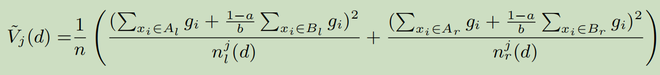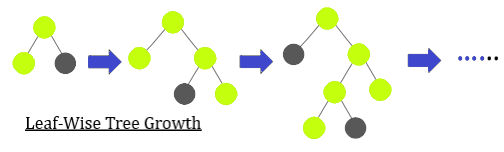# LightGBM (Light Gradient Boosting Machine)

LightGBM is a gradient boosting framework based on decision trees to increases the efficiency of the model and reduces memory usage.
It uses two novel techniques: Gradient-based One Side Sampling and Exclusive Feature Bundling (EFB) which fulfills the limitations of histogram-based algorithm that is primarily used in all GBDT (Gradient Boosting Decision Tree) frameworks. The two techniques of GOSS and EFB described below form the characteristics of LightGBM Algorithm. They comprise together to make the model work efficiently and provide it a cutting edge over other GBDT frameworks

Gradient-based One Side Sampling Technique for LightGBM:
Different data instances have varied roles in the computation of information gain. The instances with larger gradients(i.e., under-trained instances) will contribute more to the information gain. GOSS keeps those instances with large gradients (e.g., larger than a predefined threshold, or among the top percentiles), and only randomly drop those instances with small gradients  to retain the accuracy of information gain estimation. This  treatment can lead to a more accurate gain estimation than uniformly random sampling, with the same target sampling rate, especially when the value of information gain has a large range.

Algorithm for GOSS:

```Input: I: training data, d: iterations
Input: a: sampling ratio of large gradient data
Input: b: sampling ratio of small gradient data
Input: loss: loss function, L: weak learner
models ? {}, fact ? (1-a)/b
topN ? a × len(I), randN ? b × len(I)
for i = 1 to d do
preds ? models.predict(I) g ? loss(I, preds), w ? {1, 1, ...}
sorted ? GetSortedIndices(abs(g))
topSet ? sorted[1:topN]
randSet ? RandomPick(sorted[topN:len(I)],
randN)
usedSet ? topSet + randSet
w[randSet] × = fact . Assign weight f act to the
newModel ? L(I[usedSet], g[usedSet],
w[usedSet])
models.append(newModel)```

Mathematical Analysis for GOSS Technique (Calculation of Variance Gain at splitting feature j)

For a training set with n instances {x1, · · ·, xn}, where each xi is a vector with dimension s in space Xs. In each iteration of gradient boosting, the negative gradients of the loss function with respect to the output of the model are denoted as {g1, · · ·, gn}. In this GOSS method, the training instances are ranked according to their absolute values of their gradients in the descending order. Then, the top-a × 100% instances with the larger gradients are kept and we get an instance subset A. Then,  for the remaining set Ac consisting (1- a) × 100% instances with smaller gradients., we further randomly sample a subset B with size b × |Ac|. Finally, we split the instances according to the estimated variance gain at vector Vj (d) over the subset A ? B.Variance Gain using GOSS Method

where Al = {xi ? A : xij ? d}, Ar = {xi ? A : xij > d}, Bl = {xi ? B : xij ? d}, Br = {xi ? B : xij > d}, and the coefficient (1-a)/b is used to normalize the sum of the gradients over B back to the size of Ac.

Exclusive Feature Bundling Technique for LightGBM:
High-dimensional data are usually very sparse which provides us a possibility of designing a nearly lossless approach to reduce the number of features. Specifically, in a sparse feature space, many features are mutually exclusive, i.e., they never take nonzero values simultaneously. The exclusive features can be safely bundled into a single feature (called an Exclusive Feature Bundle).  Hence, the complexity of histogram building changes from O(#data × #feature) to O(#data × #bundle), while #bundle<<#feature . Hence, the speed for training framework is improved without hurting accuracy.

Algorithm for Exclusive Feature Bundling Technique:

```Input: numData: number of data
Input: F: One bundle of exclusive features
binRanges ? {0}, totalBin ? 0
for f in F do
totalBin += f.numBin
binRanges.append(totalBin)
newBin ? new Bin(numData)
for i = 1 to numData do
newBin[i] ? 0
for j = 1 to len(F) do

if F[j].bin[i] != 0 then
newBin[i] ? F[j].bin[i] + binRanges[j]
Output: newBin, binRanges```

Architecture :
LightGBM splits the tree leaf-wise as opposed to other boosting algorithms that grow tree level-wise. It chooses the leaf with maximum delta loss to grow. Since the leaf is fixed, the leaf-wise algorithm has lower loss compared to the level-wise algorithm. Leaf-wise tree growth might increase the complexity of the model and may lead to overfitting in small datasets.
Below is a diagrammatic representation of Leaf-Wise Tree Growth:Code: Python Implementation of LightGBM Model:
The data set used for this example is Breast Cancer Prediction. Click on this to get data set : Link to Data set.

 `# installing LightGBM (Required in Jupyter Notebook and  ` `# few other compilers once) ` `pip install lightgbm ` ` `  `# Importing Required Library ` `import` `pandas as pd ` `import` `lightgbm as lgb ` ` `  `# Similarly LGBMRegressor can also be imported for a regression model. ` `from` `lightgbm ``import` `LGBMClassifier ` ` `  `# Reading the train and test dataset ` `data ``=` `pd.read_csv("cancer_prediction.csv) ` ` `  `# Removing Columns not Required ` `data ``=` `data.drop(columns ``=` `[``'Unnamed: 32'``], axis ``=` `1``) ` `data ``=` `data.drop(columns ``=` `[``'id'``], axis ``=` `1``) ` ` `  `# Skipping Data Exploration ` `# Dummification of Diagnosis Column (1-Benign, 0-Malignant Cancer) ` `data[``'diagnosis'``]``=` `pd.get_dummies(data[``'diagnosis'``]) ` ` `  `# Spliiting Dataset in two parts ` `train ``=` `data[``0``:``400``] ` `test ``=` `data[``400``:``568``] ` ` `  `# Separating the independent and target variable on both data set ` `x_train ``=` `train.drop(columns ``=``[``'diagnosis'``], axis ``=` `1``) ` `y_train ``=` `train_data[``'diagnosis'``] ` `x_test ``=` `test_data.drop(columns ``=``[``'diagnosis'``], axis ``=` `1``) ` `y_test ``=` `test_data[``'diagnosis'``] ` ` `  `# Creating an object for model and fitting it on training data set  ` `model ``=` `LGBMClassifier(model ``=` `LGBMClassifier() ` `model.fit(x_train, y_train) ` ` `  `# Predicting the Target variable ` `pred ``=` `model.fit(x_test) ` `print``(pred) ` `accuracy ``=` `model.score(x_test, y_test) ` `print``(accuracy) `

```Output
Prediction array :
[0 1 1 1 1 1 1 1 0 1 1 1 1 0 1 1 1 0 1 1 1 1 1 1 1 1 1 1 1 1 0 1 0 0 1 0 1
1 1 1 1 0 1 1 0 1 0 1 1 0 1 0 1 1 1 1 1 1 1 1 0 0 1 1 1 1 1 1 0 1 1 1 1 1
1 1 1 1 1 0 1 1 1 1 1 1 1 0 1 0 1 0 0 1 1 1 1 1 0 0 1 0 1 0 1 1 1 1 1 0 1
1 0 1 0 1 0 0 1 1 1 0 1 1 1 1 1 1 1 1 1 1 1 0 1 0 0 1 1 1 1 0 0 1 1 1 1 1
1 1 1 1 1 1 1 1 1 1 1 1 1 1 0 0 0 0 0 0]
Accuracy Score :
0.9702380952380952
```

Parameter Tuning
Few important parameters and their usage is listed below :

1. max_depth : It sets a limit on the depth of tree. The default value is 20. It is effective in controlling over fitting.
2. categorical_feature : It specifies the categorical feature used for training model.
3. bagging_fraction : It specifies the fraction of data to be considered for each iteration.
4. num_iterations : It specifies the number of iterations to be performed. The default value is 100.
5. num_leaves : It specifies the number of leaves in a tree. It should be smaller than the square of max_depth.
6. max_bin : It specifies the maximum number of bins to bucket the feature values.
7. min_data_in_bin : It specifies minimum amount of data in one bin.
8. task : It specifies the task we wish to perform which is either train or prediction. The default entry is train. Another possible value for this parameter is prediction.
9. feature_fraction : It specifies the fraction of features to be considered in each iteration. The default value is one.

Attention reader! Don’t stop learning now. Get hold of all the important CS Theory concepts for SDE interviews with the CS Theory Course at a student-friendly price and become industry ready.

My Personal Notes arrow_drop_upCheck out this Author's contributed articles.

If you like GeeksforGeeks and would like to contribute, you can also write an article using contribute.geeksforgeeks.org or mail your article to contribute@geeksforgeeks.org. See your article appearing on the GeeksforGeeks main page and help other Geeks.

Please Improve this article if you find anything incorrect by clicking on the "Improve Article" button below.